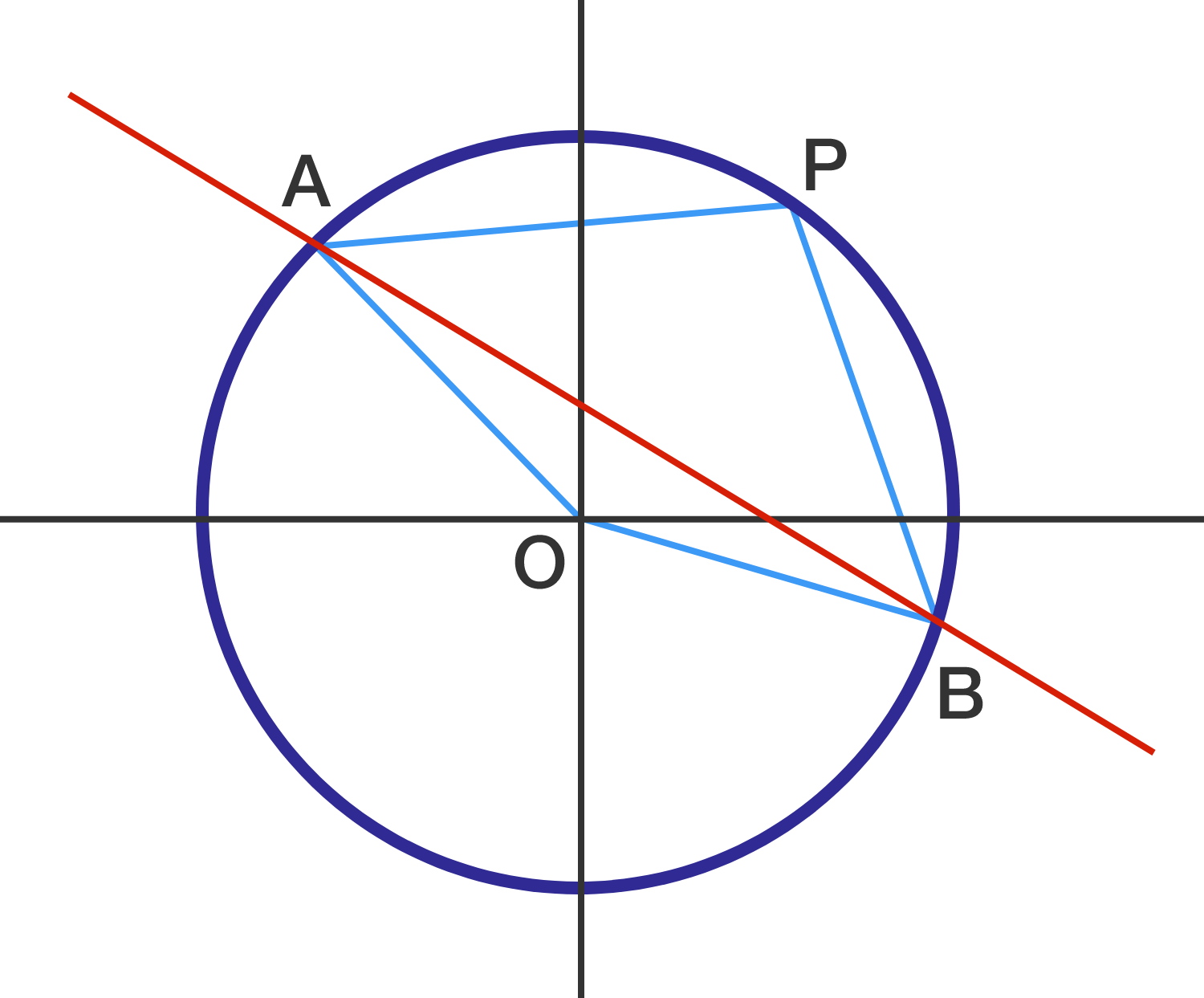# Cut Through the Circle

Geometry Level 1Circle $O$ is centered at the point of origin with point $P = (3 , 4)$ lying on it. The red line $l : 3x + 4y - 7 = 0$ intersects the circle at points $A$ and $B,$ as shown.

What is the area of quadrilateral $AOBP?$

×